# Logarithms: equations, systems and properties

Author:
JLF

## 1. Properties

1. Logarithm of a product﻿ 2. Logarithm of a quotient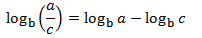﻿ 3.  Logarithm of a power﻿ 4. Change of base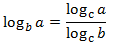﻿ 5. Inverse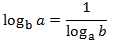## 2. Equations

logarithmic equation is an equation that has an unknown factor in the argument of a logarithm. In reality, the resolution is reduced to the resolution of equations of the same type as the expressions in the arguments (quadratic equations, cubic equations, irrational equations...).
Example 1We will use the properties of logarithms and that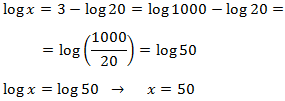﻿ We have an equality between logarithms, so the arguments (what is inside) has to be the same: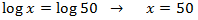﻿ The solution to the equation is x = 50.
Example 2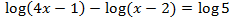﻿## 3. Logrithmic equation systems

Example 1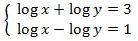﻿ We apply the change of variable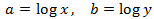﻿ This way we obtain the following linear equation system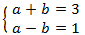﻿ we resolve it and we undo the cange of variable: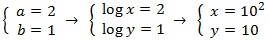Example 2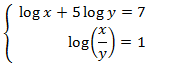﻿ First we apply the property logarithm of a product:﻿ We apply the change of variable:﻿ and we obtain the following system: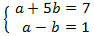﻿ that has as solution: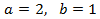﻿ Finally, we undo the change of variable: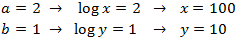﻿ We do not need to check the solutions.
More examples: